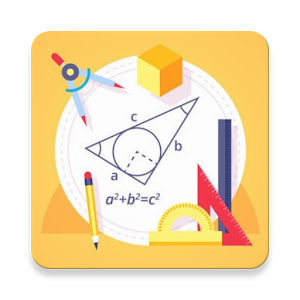# Math - complete pocket guide - Lumos Educational App Store4.61
Price -
\$

#### DESCRIPTION:

Best collection of math formulas with explanation! Particularly useful for students who is going to pass math exams. The app covers: ALGEBRA Sets of Real Numbers Properties of Real Numbers Properties of Equality Properties of Fractions Division Properties of Zero Real Number Line Interval Absolute Value Distance between Two Points on the Number Line Definition of Positive Integer Exponents Definition of bâ° Definition of bâ»â¿ Properties of Exponents Definition of â¿âa Properties of Radicals General Form of a Polynomial Factoring Polynomials Orde

#### OVERVIEW:

Math - complete pocket guide is a free educational mobile app By Study Apps.It helps students in grades HS practice the following standards HSN.RN.A.1,HSN.RN.A.2,HSN.RN.B.3.

This page not only allows students and teachers download Math - complete pocket guide but also find engaging Sample Questions, Videos, Pins, Worksheets, Books related to the following topics.

1. HSN.RN.A.1 : Explain how the definition of the meaning of rational exponents follows from extending the properties of integer exponents to those values, allowing for a notation for radicals in terms of rational exponents. For example, we define 51/3 to be the cube root of 5 because we want (51/3)3 = 5(1/3)3 to hold, so (51/3)3 must equal 5.

2. HSN.RN.A.2 : Rewrite expressions involving radicals and rational exponents using the properties of exponents..

3. HSN.RN.B.3 : Explain why the sum or product of two rational numbers is rational; that the sum of a rational number and an irrational number is irrational; and that the product of a nonzero rational number and an irrational number is irrational.

HS

HSN.RN.A.1
HSN.RN.A.2
HSN.RN.B.3
HSN.CN.A.1
HSN.CN.A.2
HSN.CN.A.3

#### ADDITIONAL INFORMATION:

Developer: Study Apps

Software Version: 1.1.2

Category: Education

### RELATED APPS